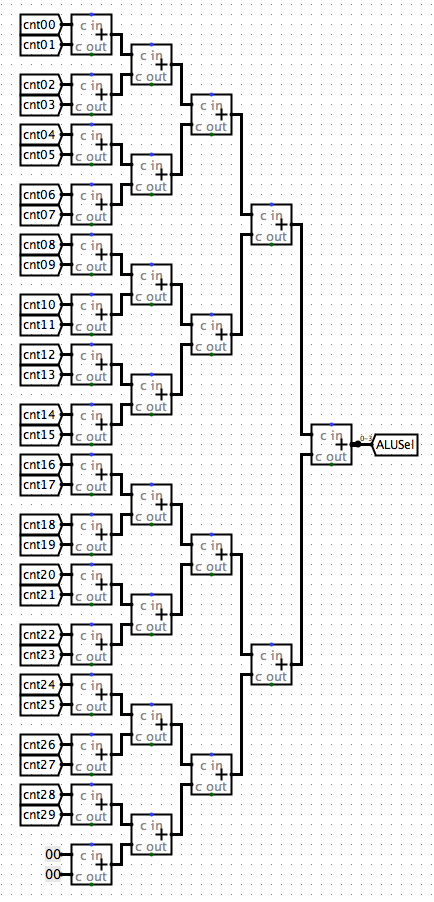# Shixuan Li

0%

Decoding and ALU Selection

## 3. Instruction Analysis

We assume that you have basic understanding about RISC-V, if you are not so familiar with RISC-V, please click HERE(not published) to see my related article.

Each type of RISC-V instructions has an special encoding format to convert the assembly scripts into machine codes. Here, given the machine codes, we need to determine which instruction it is by comparing with the formatting regulations.

### 3.1 Breaking the Instructions

To determine the type and value information of each type, we need to cut off certain parts of the instruction to do comparison. Thus, we want to first get all the possible information so that it will be much convenient in our further application.

We use splitters to get sections of information from the instruction

According to the formats shown above, we need to break the instruction based on bit positions from bit 31 to bit 0 and get sections. However, we do not have to do it for every type, since there are some common parts for the decoding. For example, for `Rtype` we need to decode the instruction into `func7`, `rs2`, `rs1`, `func3`, `rd`, and `opcode`.

Note that since the position of `opcode`, `rs2`, `rs1`, `rd` are the same for all types (if needed), we do not need to replicate doing these parts.

And we can realize that for other parts, we just need to deal with the `immediate` parts.

The full version of first-stage decoding is presented as following

### 3.2 Determining the Types

As part of the RISC-V knowledge, we know that we should use `opcode` to determine the type of instruction. Also according to the Instructions Sheet at the very top of this page, we can do our analyzing by performing:

Note that since the specialty of the `Itype`, we break the `Itype` into three categories which are `Itype-Load`, `Itype-Other`, and `Itype-Jalr`.

### 3.3 Determining the Instruction

Congrats! You are at the most tedious part in this CPU. According to the Instruction Sheet, we need to consider over `opcode`(Type), `func3`, and `func7` in order to determine the exact instruction.

### 3.4 ALU Selection

Now we've successfully decoded the instructions. What's next?

#### 3.4.1 Intuition

Note that we need to implement the corresponding ALU unit according to our result. How can we make this happen? Note that ALU is set of arithmetic units, with the given input. Thus, we need to make an index in our control panel to give our ALU a signal noticing which arithmetic unit we are using.

The solution to this is that we use an Multiplexer in our ALU unit (more details in Part 5), and we will create an controller `AluSel` to help choose the correct arithmetic unit. There are totally 13 arithmetic units we will implement for this CPU. Thus, we need to use a 4 bit Multiplexer.

To do this, we convert our decoding results into bit-representation. To be more specific, for example, we assign `add` unit to `0000` and `and` to `0001` .....

#### 3.4.2 Implementation

##### 3.4.2.1 Indicator

How can we make this happen? The intuition here is that since each instruction will only be decoded as one implementation, we assign value to each kind of implementation. There are totally 30 kinds of instructions. We first assign 30 different indexes (denoted as `ctrxx`) to indicate if the instruction is corresponding to the implementation it represents.

##### 3.4.2.2 Counting

Then, we using each `ctrxx` as an controller for a Multiplexer, getting corresponding `cntxx` as `0` if the instruction does not correspond to the instruction it represents, and `xxx`(the index number of arithmetic unit we need to implement considering the instruction, note that we should set the instructions that will use the same kind of arithmetic unit).

Here I follow the following indexing of ALU system

Now we are at the final step. We will accumulate the values of the indexes and store the result in `ALUSel`. The intuition here is that since each instruction has unique type of implementation type, there will be only one result of the counters to be non-zero, which indicates which arithmetic unit we are using. This way, we are able to figure out an signal to out ALU, telling it which arithmetic unit to implement.
The accumulation look like this;In Part 4 we will talk about the `RegFile` unit of the CPU, which implement the registers, writing and reading data from the registers.